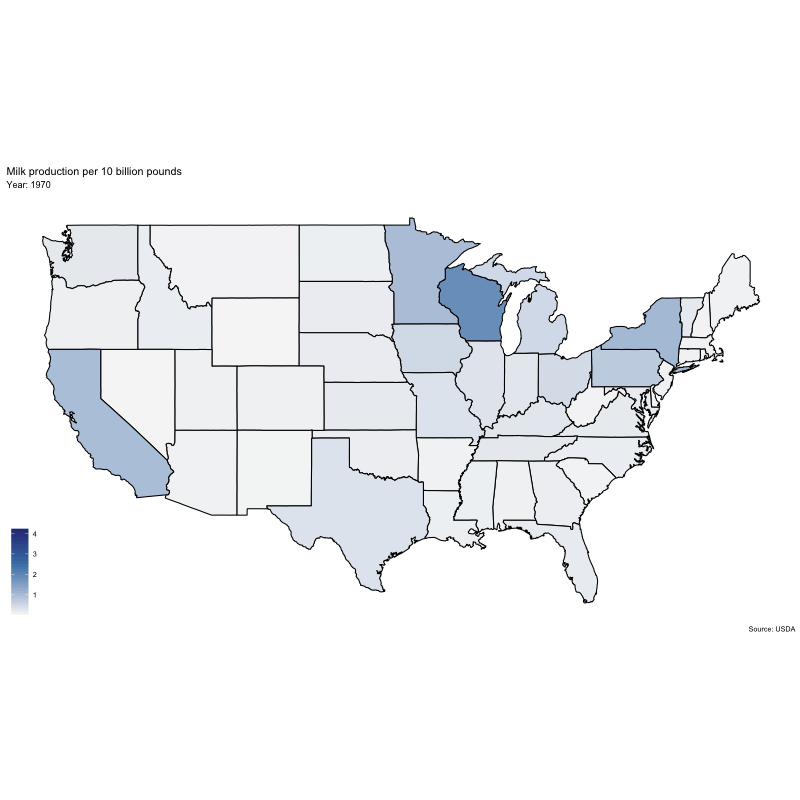Analyzing data for #tidytuesday week of 1/29/2019 (source)

``````# LOAD PACKAGES
library(tidyverse)
library(scales)
library(lubridate)
library(ggmap)
library(gganimate)
library(ggthemes)
library(transformr)
library(gifski)
library(mapproj)

milk <- milk_raw``````

### Extract geospatial data and parse data

``````usa <- as_tibble(map_data("state"))
usa\$region <- str_to_title(usa\$region)
usa <- usa %>%
rename(state = region)

milk_parsed <- milk %>%
select(-region) %>%
mutate(milk_10billion = milk_produced / 10000000000,
year = as.integer(year)) %>%
full_join(usa) %>%
filter(!is.na(year), !is.na(long), !is.na(lat))``````

### Build animation

``````milk_animation <- milk_parsed %>%
ggplot(aes(long, lat, group = group, fill = milk_10billion)) +
geom_polygon(color = 'black') +
scale_fill_gradient2(low = "gray97", mid = "steelblue", high = "midnightblue", midpoint = 2.5) +
theme_map() +
coord_map() +
labs(x = NULL,
y = NULL,
fill = NULL,
title = "Milk production per 10 billion pounds",
subtitle = "Year: {round(frame_time)}",
caption = "Source: USDA") +
transition_time(year)

animate(milk_animation, height = 800, width = 800)``````